Open in App
Not now

# When to use useCallback, useMemo and useEffect ?

• Difficulty Level : Medium
• Last Updated : 26 Jul, 2021

The useCallback, useMemo, and useEffect are a way to optimize the performance of React-based applications between rerendering of components. These functions provide some of the features of the class-based components like persistence of dedicated states through render calls as well as the lifecycle functions to control how the components look over various stages of their lifecycle.

To answer when to use useCallBack, useMemo, and useEffect, we should know what exactly they do and how they are different.

1. useCallback: The useCallback is a react hook that returns a memoized callback when passed a function and a list of dependencies as parameters. It’s very useful when a component is passing a callback to its child component to prevent the rendering of the child component. It only changes the callback when one of its dependencies gets changed.

2. useMemo: The useMemo is similar to useCallback hook as it accepts a function and a list of dependencies but it returns the memoized value returned by the passed function. It recalculated the value only when one of its dependencies change. It is useful to avoid expensive calculations on every render when the returned value is not going to change.

3. useEffect: A hook that helps us to perform mutations, subscriptions, timers, logging, and other side effects after all the components has been rendered. The useEffect accepts a function that is imperative in nature and a list of dependencies. When its dependencies change it executes the passed function.

Creating a react application for understanding all the three hooks:

• Step 1: Create a React application using the following command:

`npx create-react-app usecallbackdemo`
• Step 2: After creating your project folder i.e. foldername, move to it using the following command:

`cd usecallbackdemo`

Project Structure: It will look like the following.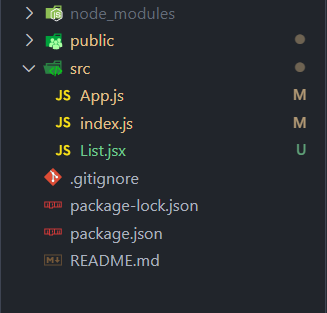The project structure

Now let’s understand the working of all three hooks.

1. usecallback: It depends on referential equality. In javascript, functions are first-class citizens, meaning that a function is a regular object. Hence, two function objects even when they share the same code are two different objects. Just remember that a function object is referentially equal only to itself.

Let’s see this in the following code, doubleFactory creates and returns a function:

## Javascript

 `function` `doubleFactory(){``    ``return` `(a) => 2*a;``}`` ` `const double1 = doubleFactory();``const double2 = doubleFactory();`` ` `double1(8); ``// gives 16``double2(8); ``// gives 16`` ` `double1 === double2;  ``// false``double1 === double1;  ``// true`

doube1 and double2 doubles the value passed to them and are created by the same factory function. The two functions even when they share the same code, are not equal, here (double1 === double2) evaluates to false.

When to use useCallback: In React, a component usually has some callback function created within it.

## Javascript

 `function` `MyComponent(){`` ` `    ``// HandleChange is created on every render``    ``const handleChange = () => {...};``     ` `    ``return` `<> ``        ``... ``        ``;``}`

Here handleChange function objects are different on every rendering of MyComponent. And there are several cases when we may want the same function object between multiple renderings. For example when it’s a dependency for some other hooks (useEffect( …, callbackfunc)) or when the function object itself has some internal state that we need to maintain. In such a case, useCallback hook comes handy. In simple words, useCallback( callBackFun, deps ) returns a memorized callback when the dependency values deps do not change between renderings. (memoized here refers to caching the object for future use).

Let’s see a use case using a project: The application consists of an input field, a button, and a list. The list is a component that consists of two numbers, the first is input plus 10 and the second is input + 100. The button changes the components from dark mode to light mode and vice versa.

There’s going to be two components App and List, App is our main component where we add input field, button, and List. The list component is used to print the list of items depending on the input field.

## App.jsx

 `import React, { useState} from ``"react"``import List from ``"./List"`` ` `function` `App(){`` ` `    ``{``/* Initial states */``}``    ``const [input, setInput] = useState(1);``    ``const [light, setLight] = useState(``true``);`` ` `    ``{``/* getItems() returns a list of number which``    ``is number+10 and number + 100 */``}``    ``const getItems = () => {``        ``return` `[input + 10, input + 100];``    ``}`` ` `    ``{``/* Style for changing the theme */``}``    ``const theme = {``        ``backgroundColor: light ? ``"White"``: ``"grey"``,``        ``color: light ? ``"grey"` `: ``"white"``    ``}``    ` `    ``return` `<>``        ``{``/* set the theme in the parent div */``}``        ``
``            `` setInput(parseInt(event.target.value))} />`` ` `            ``{``/* on click the button the theme is set to the ``            ``opposite mode, light to dark and vice versa*/``}``            ````            ````        ``
``    ``;``}`` ` `export ``default` `App;`

## List.jsx

 `import React, { useEffect, useState } from ``"react"`` ` `function` `List({ getItems }){`` ` `    ``/* Initial state of the items */``    ``const [items, setItems] = useState([]);`` ` `    ``/* This hook sets the value of items if ``       ``getItems object changes */``    ``useEffect(() => {``        ``console.log(``"Fetching items"``);``        ``setItems(getItems());``    ``}, [getItems]);`` ` `    ``/* Maps the items to a list */``    ``return` `
``        ``{items.map(item =>
{item}
)}``    ``
``}``export ``default` `List;`

Explanation: The list component gets the getItems function as a property. Every time the getItems function object changes useEffect will call setItems to set the list returned from the function object to stateful variable items and then we map those items into a list of div.Every time items are fetch using getItems in useEffect, we print “Fetching items” to see how often the items are fetched.

Step to run the application:

`npm start`

Output: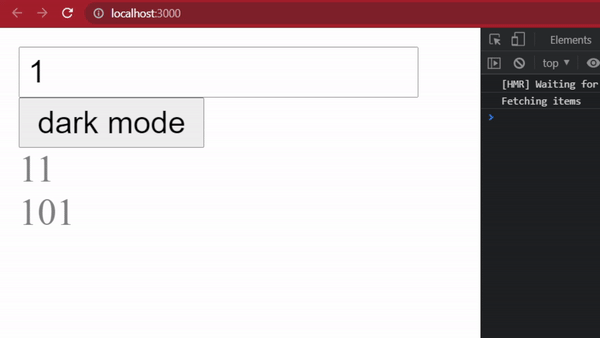Explanation: The following will be the output when a user enters a number into the input field. It can be seen from the console log that when the app is rendered for the first time, items are fetched, and “fetching items” are printed. Now if we input some different numbers, we see that items are fetched once again.

Now the weird thing is, when we press the button to change the theme, we see that the items are still being fetched even when the input field is not modified.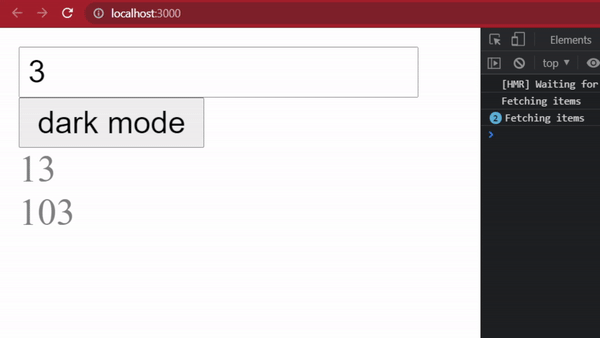The reason behind this behavior is that when we press the button, the app component is rerendered, and hence the function getItems() inside App is again created and we know that two objects are referentially different. Hence, inside the List component, useEffect hook calls the setItems and prints “Fetching items” as its dependency has changed.

The solution to the above problem: Here we can use the useCallback function to memoise the getItems() function depending upon the input number. We don’t want to recreate the function unless the input changes, and hence, on pressing the button (changing the theme) items will not be fetched.

## App.jsx

 `import React, { useCallback, useState} from ``"react"``import List from ``"./List"`` ` `function` `App(){`` ` `    ``{``/* Initial states */``}``    ``const [input, setInput] = useState(1);``    ``const [light, setLight] = useState(``true``);`` ` `    ``{``/* useCallback memoizes the getItems() which ``       ``returns a list of number which is number+10``       ``and number + 100 */``}``    ``const getItems = useCallback(() => {``        ``return` `[input + 10, input + 100];``    ``}, [input]);`` ` `    ``{``/* style for changing the theme */``}``    ``const theme = {``        ``backgroundColor: light ? ``"White"``: ``"grey"``,``        ``color: light ? ``"grey"` `: ``"white"``    ``}``     ` ` ` `    ``return` `<>``        ``{``/* set the theme in the parent div */``}``        ``
``            `` ``            ``setInput(parseInt(event.target.value))``            ``} />`` ` `            ``{``/* on click the button the theme is set to ``            ``the opposite mode, light to dark and vice versa*/``}``            ````            ````        ``
``    ``;``}`` ` `export ``default` `App;`

Now we use the useCallback hook to memoize the getitems function which takes the function and a dependency list. The dependency list in our case includes only the input.

Output: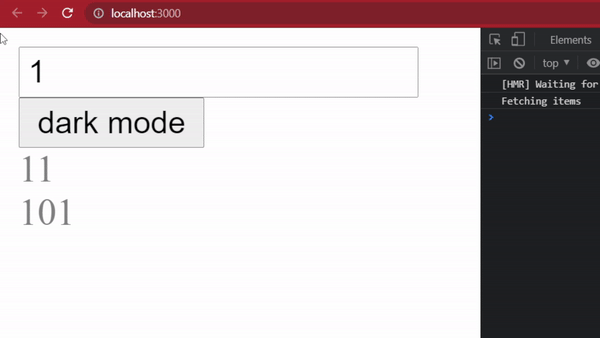Explanation: It can be seen from the output that the items are fetched only once when the app is rendered but not when we change the theme by pressing the button. It does not matter how many times we flip the theme, useEffect will not call the setItems until the input field has a new number.

2. useMemo: The useMemo hook returns a memoised value after taking a function and a list of dependencies. It returns the cached value if the dependencies do not change. Otherwise, it will recompute the value using the passed function.

When to use useMemo:

There are two cases where using useMemo can be helpful:

1. When a component uses a value computed using a time-consuming function.

## MyComponent.jsx

 `function` `MyComponent(){``    ``const [data, setData] = useState(0);``    ``const number = verySlowFunction(data);``    ``return` `
{number}
;``}`` ` `function` `verySlowFunction(input){``    ``...heavy work done here``    ``return` `value;``}`

Here the slow function is called every time MyComponent is rendered, maybe because some stateful variable is changed or some other component caused the rerendering.

Solution: By memoizing the returned value of the slow function using the useMemo hook we can save ourselves from the delay it may cause.

## MyComponent.jsx

 `function` `MyComponent(){``    ``const [data, setData] = useState(0);``    ``const number = useMemo(() => {``        ``return` `verySlowFunction(data)}, [data]);``     ` `    ``return` `
{number}
;``}`` ` `function` `verySlowFunction(input){``    ``...heavy work done here``    ``return` `value;``}`

here we use the useMemo hook to cache the returned value and the dependency list contains the data stateful variable. Now every time the component is rendered, if the data variable is not altered, we get the memoized value without calling the CPU intensive function. Hence, it improves the performance.

2. Now consider another scenario when we have a component that does something when some data changes, for example, a let’s take the hook useEffect which logs if some dependency changes.

## MyComponent.jsx

 `function` `MyComponent() {    ``    ``const [number, setNumber] = useState(0);``    ``const data = {``        ``key: value``    ``};``     ` `    ``useEffect(() => {``        ``console.log(``'Hello world'``);``    ``}, [data]);``}`

In the above code, every time the component is rendered, “Hello world” is printed on the console due to the fact that the data object that is stored in the previous render is referentially different in the next render and hence the useEffect hook runs the console.log function. In real-world useEffect can contain some functionality that we don’t want to be repeated if its dependencies do not change.

Solution: We can memoize the data object using the useMemo hook so that rendering of the component won’t create a new data object and hence useEffect will not call its body.

## MyComponent.jsx

 `function` `MyComponent(){``     ` `    ``const [number, setNumber] = useState(0);`` ` `    ``const data = useMemo( () => {``    ``return` `{``        ``key: value``    ``}}, number);``     ` `    ``useEffect(() => {``        ``console.log(``'Hello world'``);``    ``}, [data]);``}`

Now when the component renders for the second time and if the number stateful variable is not modified then console.log() is not executed.

3. useEffect: In react, side effects of some state changes are not allowed in functional components. To perform a task once the rendering is complete and some state changes, we can use useEffect. This hook takes a function to be executed and a list of dependencies, changing which will cause the execution of the hook’s body.

To understand its proper use. let’s see a simple example:

Example: Consider a scenario where we have to fetch some data from some API once the components are mounted. In the example code, we simulate the server with our data object with values of different colours and fruits. We want to print the list of items depending on the button being pressed. Hence we have two state variables currentChoice and items which are modified by pressing the buttons. When a button is pressed it changes the currentChoice and the body of useEffect is called and current choice’s items are printed using a map. Now if we don’t use useEffect, every time a button is pressed data will be fetched from the server even if the choice does not change. In such a condition this hook helps us to not call the fetching logic unless our choice changes.

## App.jsx

 `import React, { useEffect, useState} from ``"react"`` ` ` ` `function` `App(){`` ` `    ``/* Some data */``    ``const data = {``        ``Colors: [``"red"``, ``"green"``, ``"yellow"``],``        ``Fruits: [``"Apple"``, ``"mango"``, ``"Banana"``]``    ``}`` ` `    ``/* Initial states */``    ``const [currentChoice, setCurrentChoice] = useState(``"Colors"``);``    ``const [items, setItems] = useState([]);`` ` `    ``/* Using useEffect to set the data of currentchoice``       ``to items and console log the fetching... */``    ``useEffect(() => {``        ``setItems(data[currentChoice]);``        ``console.log(``"Data is fetched!"``);``    ``}, [currentChoice]);``     ` `    ``return` `<>``    ````    ``    ``        ``{items.map(item => {``return` `
{item}
})}``    ``;``}`` ` `export ``default` `App;`

Output: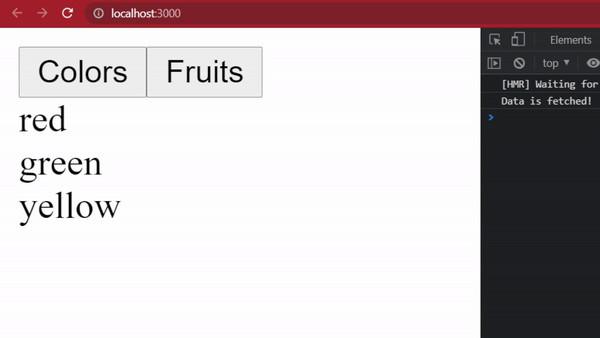Explanation:  When the application loads for the first time, data is fetched from our fake server. This can be seen in the console in the image below. And when we press the Fruits button, appropriate data is again fetched from the server and we can see that “Data is fetched” is again printed in the console. But if we press the Fruits button again, we don’t have to get the data from the server again as our choice state does not change.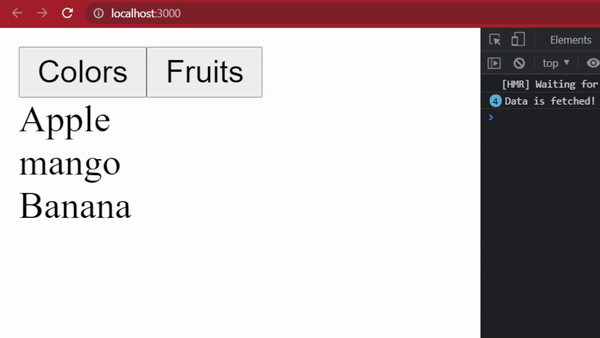Conclusion:

Hence, a useCallback hook should be used when we want to memoize a callback, and to memoize the result of a function to avoid expensive computation we can use useMemo. useEffect is used to produce side effects to some state changes.One thing to remember is that one should not overuse hooks.

My Personal Notes arrow_drop_up